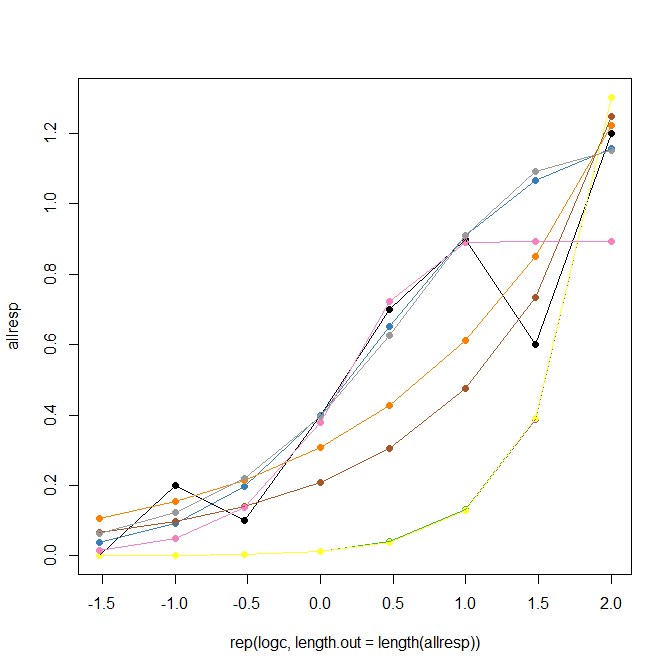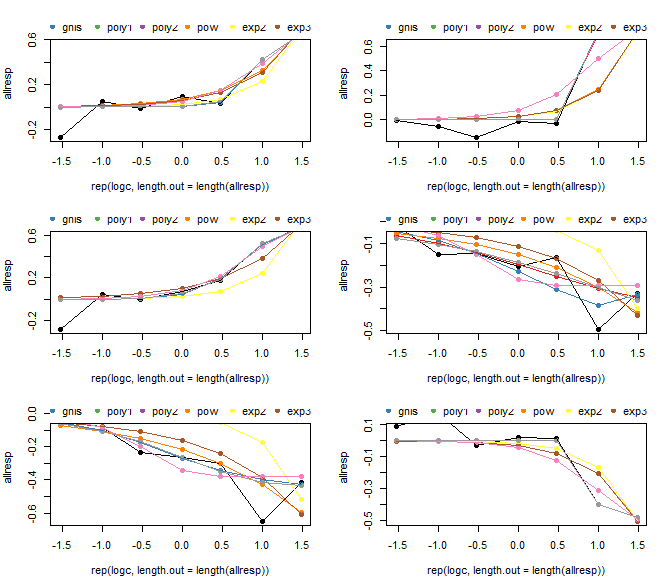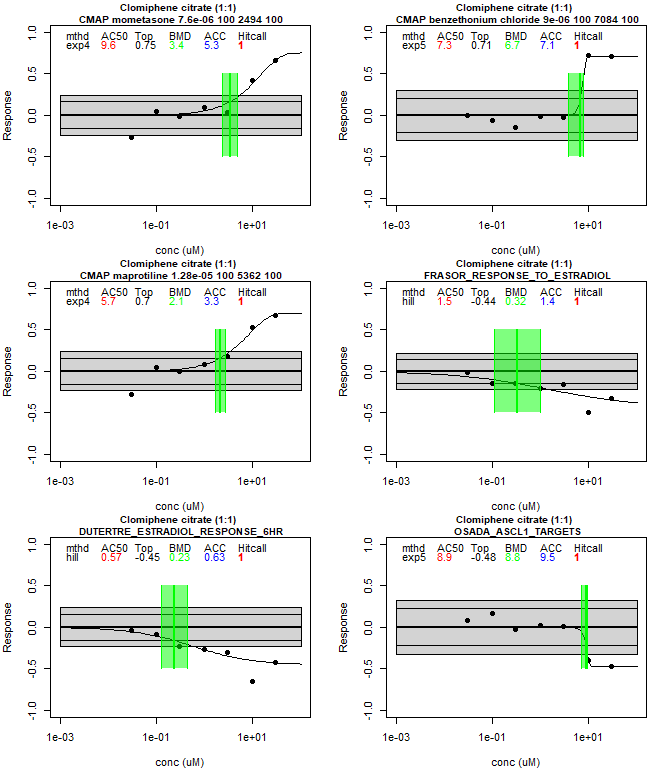# tcplfit2-vignette

library(tcplfit2)

# Getting started with tcplfit2

The package tcplfit2 contains the core concentration-response functionality of the package tcpl (The ToxCast Pipeline) built to process all of the ToxCast high-throughput screen (HTS) data at the US EPA. Much of the rest of the code in tcpl is used to do data processing, normalization, and database storage. We wanted to reuse the core concentration-response code for other projects, and add extensions to it, which was the origin of the current package tcplfit2. The main set of extensions was to include all of the concentration-response models that are contained in the program BMDExpress. These include exponential and power functions in addition to the original Hill, gain-loss and constant models. Additionally, we wanted to include BMD (Benchmark Dose Modeling) outputs, which is simply defining a Benchmark Response (BMR) level and setting the BMD to the concentration where the curve crosses the BMR level. One final addition was to let the hitcall value be a continuous number ranging from 0 to 1. This vignette describes the basic functionality of this package with two simple examples.

## Example 1 - Running a single concentration-response calculation

All calculations use the function concRespCore which has several key inputs. The first set are put into a named list called ‘row’:

• conc - a vector of concentrations (not log concentrations)
• resp - a vector of responses, of the same length as conc. Note that replicates are allowed, i.e. there can be multiple pairs of conc and resp with the same concentration value.
• cutoff- this is the value that the response must exceed before a a curve can be called a hit. For ToxCast, this is usually some multiple (typically 3) of the median absolute deviation (BMAD) around baseline for the lowest two concentration. The user is free to make other choices
• bmed - this is the median of the baseline, and will usually be set to zero. If not, the entire response series will be shifted by this amount.
• onesd- This is one standard deviation of the nose around the baseline. The BMR value = onesd*bmr_scale. The default bmr_scale is 1.349.

The list row can also have other optional elements which will be included in the output. These can be, for instance, the name of the chemical (or other identifiers) or the name of the assay being modeled. Two other parameters might be used. The first is a Boolean conthits. If TRUE (the default, and recommended usage), the hitcall returned will be a continuous value between 0 and 1. The other is do.plot. If this is set to TRUE (default is FALSE), a plot of the curve will be generated. The user can also select only a subset of the models to be run. The example below has all of the possible ones included. the model cnst always needs to be included. For some applications, we exclude the gnls model.

To run a simple example, use the following code …

  conc <- list(.03,.1,.3,1,3,10,30,100)
resp <- list(0,.2,.1,.4,.7,.9,.6, 1.2)
row = list(conc = conc, resp = resp, bmed = 0, cutoff = 1, onesd = .5,name="some chemical")
res <- concRespCore(row,fitmodels = c("cnst", "hill", "gnls", "poly1", "poly2", "pow", "exp2", "exp3",
"exp4", "exp5"),conthits = T, do.plot=T)The output of this run will be a data frame with one row, summarizing the results for the winning model.

## Example 2: Running a series of concentration-response models for a single assay

The input data for this example is taken from one of the Tox21 HTS assays, for estrogen receptor (ER) agonist activity. The data is from the mc3 table in the database invitrodb, which is the back end for tcpl. This example will run 6 chemicals out of the 100 that are included in the data set, and will create plots for these. The plotting routine concRespPlot is somewhat generic, and we anticipate that users will make their own version of this. To run this example, use the following code …

  # read in the data
file <- "data/mc3.RData"

# set up a 3 x 2 grid for the plots
on.exit(par(oldpar))
par(mfrow=c(3,2),mar=c(4,4,2,2))

# determine the background variation
temp <- mc3[mc3$logc<= -2,"resp"] bmad <- mad(temp) onesd <- sd(temp) cutoff <- 3*bmad # select six samples. Note that there may be more than one sample processed for a given chemical spid.list <- unique(mc3$spid)
spid.list <- spid.list[1:6]

for(spid in spid.list) {
# select the data for just this sample
temp <- mc3[is.element(mc3$spid,spid),] # The data file has stored concentration in log10 form, so fix that conc <- 10**temp$logc
resp <- temp\$resp

# pull out all of the chemical identifiers and the name of the assay
dtxsid <- temp[1,"dtxsid"]
casrn <- temp[1,"casrn"]
name <- temp[1,"name"]
assay <- temp[1,"assay"]

# create the row object
row <- list(conc = conc, resp = resp, bmed = 0, cutoff = cutoff, onesd = onesd,assay=assay,dtxsid=dtxsid,casrn=casrn,name=name)

# run the concentration-response modeling for a single sample
res <- concRespCore(row,fitmodels = c("cnst", "hill", "gnls", "poly1", "poly2", "pow", "exp2", "exp3",
"exp4", "exp5"),conthits = T, aicc = F,bidirectional=F)

# plot the results
concRespPlot(res,ymin=-10,ymax=100)
}

One would typically save the result rows in a data frame end export these for further analysis. You could remove the plotting function from the current loop and have a loop that read from the overall results data frame and only plot selected results (e.g. those with significant responses).

## Example 3: Plotting concentration-response modeling on transcriptional signatures

The input data for this example contains 6 signatures for one chemical in a transcriptomics data set. This data set is a sample from the signature scoring method that provides the cutoff, one standard deviation, and the concentration-response data. The example illustrates two kinds of plots available in tcplfit2. In the call to concRespCore(), the argument do.plot is set to TRUE, which provides a simple plot showing results of all the different curve fitting methods. Next, utilizing the function concRespPlot() provides a more informative plot for the winning model.

  # call additional R packages
library(stringr)  # string management package

data("signatures")

# set up a 3 x 2 grid for the plots
on.exit(par(oldpar))
par(mfrow=c(3,2),mar=c(4,4,2,2))

# fit 6 observations in signatures
for(i in 1:nrow(signatures)){
# set up input data
row = list(conc=as.numeric(str_split(signatures[i,"conc"],"\\|")[]),
resp=as.numeric(str_split(signatures[i,"resp"],"\\|")[]),
bmed=0,
cutoff=signatures[i,"cutoff"],
onesd=signatures[i,"onesd"],
name=signatures[i,"name"],
assay=signatures[i,"signature"])
# run concentration-response modeling (1st plotting option)
out = concRespCore(row,conthits=F,do.plot=T)
if(i==1){
res <- out
}else{
res <- rbind.data.frame(res,out)
}
}# set up a 3 x 2 grid for the plots
# plot results using concRespPlot(2nd plotting option)
}